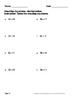## One Step Equations - Multiplication & Division Worksheets\$4.00Buy This Resource Now

One Step Equations - Multiplication and Division Worksheets.
Package includes 150 worksheets.
Answers included.

Table of Contents.
One-Step Equations - Multiplication (50 Worksheets)
Example:
x * 2 = 6

One-Step Equations - Division (50 Worksheets)
Example:
x ÷ 2 = 4
10 ÷ x = 5

One-Step Equations - Multiplication & Division (50 Worksheets)
Example:
4x = 8
x ÷ 2 = 4
6 ÷ x = 8

You can purchase all One-Step Equations Operations Worksheets (Addition, Subtraction, Multiplication and Division Worksheets) and save your money!
One-Step Equations 6\$

----------------------------------

You May Also Like These Worksheets:
One-Step Equations - All Basic Operations
One-Step Equations - Addition & Subtraction
One-Step Equations - Multiplication & Division
Two-Step Equations

You May Also Like These Task Cards:
One Step Equations Task Cards
Two Step Equations Task Cards

Be the first to know about my new discounts, freebies and product launches:
- Look for the green star next to my store logo and click it to become a follower. You will now receive email updates about this store.
- Follow me on Facebook
- Follow me on Pinterest

File Type:
PDF (Acrobat) Document File
Be sure that you have an application to open this file type before downloading and/or purchasing.
302 pages